# Basic Concepts of Inverse Trigonometric Functions

#### description

• sine, cosine, tangent, cotangent, secant, cosecant function

#### notes

In Class XI, we have studied trigonometric functions, which are defined as follows:

 Trigonometric functions Domain Range sin R [-1,1] cos R [-1.1] tan R- {x:x= (2n+1)π/2,  n∈ Z} R cot R- {x:x= nπ, n∈ Z} R sec R- {x:x= (2n+1) π/2, n∈ Z} R- (-1,1) cosec R- {x:x= nπ, n∈ Z} R- (-1,1)

We already know that, if f: x→y
f(x)= y and f is bijective,
then there exists g: y→x
g(y)= x
g is called "f"^-1
So, with the help of this knowledge we will find the inverse of the trigonometric functions
1)Inverse of sin-
Sin R→[-1,1]
sin x= y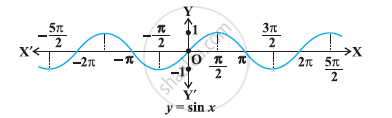sin: [-π/2, π/2]→[-1,1]

sin^(-1): [-1,1]→[-π/2, π/2], here [-π/2, π/2] is the principle range

sin^(-1)y= x

2) Inverse of cos-
cos: R→[-1,1]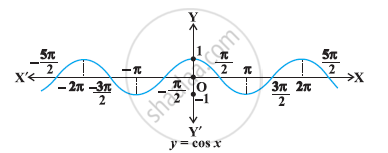cos: [0, π]→[-1,1]
cos (x)=y is a bijective function
cos^(-1): [-1,1]→[0,π], here [0,π] is the principle range
cos^-y=x
3) Inverse of tan-
tan: "R"- {x:x= (2n+1)π/2,  n∈ "Z"}→"R"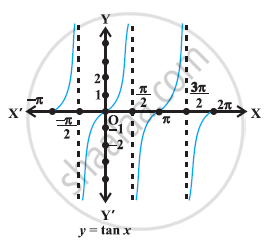tan: [(-π/2),  (π/2)]→"R"

tan x= y is a bijective function

"tan"^(-1): R→(-pi/2, pi/2), where (-pi/2, pi/2) is the principle range.

tan^(-1)y= x

4) Inverse of cot-
"cot": "R"- {x:x= n pi, n∈"Z"}→R}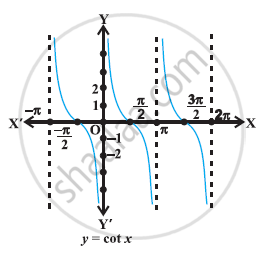cot: (0, pi)→"R"
cot x= y is a bijective function
cot ^(-1):" R"→(0, pi) , where (0, pi) is the principle range.
cot :"R"→ (0, pi)
cot^(-1)y= x
5) Inverse of sec-
sec: "R"-{x:x= (2n+1) pi/2}→"R"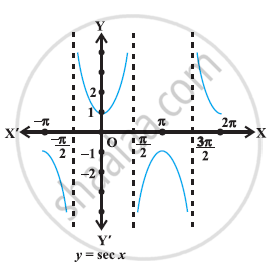sec: [0, pi]- {pi/2}→"R"- (-1,1)

sec x= y is bijective function

sec^-1: "R"- (-1,1) →[0, pi]- {pi/2}, where [0, pi]- {pi/2} is the principle range

6) Inverse of cosec-
cosec: "R"- {x=n pi, n∈"Z"}→"R"-(-1,1)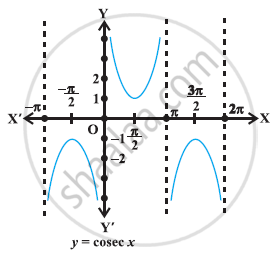cosec: [-pi/2, pi/2] -{0}→"R"-(-1,1)

cosec x= y is bijective function

cosec^-1: "R"-(-1,1)→[-pi/2, pi/2]-{0}, where [-pi/2, pi/2] -{0} is the principle range.

The following table gives the inverse trigonometric function (principal value branches) along with their domains and ranges.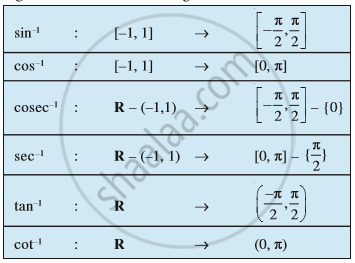If you would like to contribute notes or other learning material, please submit them using the button below.

#### Video Tutorials

We have provided more than 1 series of video tutorials for some topics to help you get a better understanding of the topic.

Series 1

Series 2

Series 3

### Shaalaa.com

Inverse Trigonometry Functions part 2 (Natural domain Range) [00:04:24]
S
0%